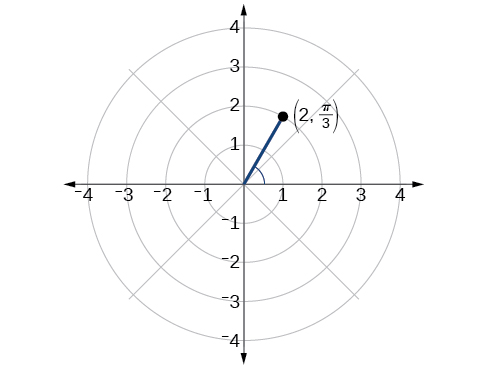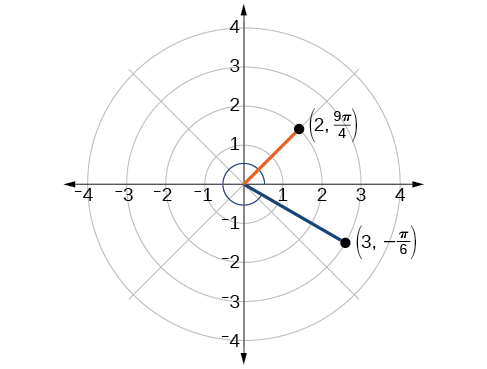# 10.3 Polar coordinates

 Page 1 / 8
In this section, you will:
• Plot points using polar coordinates.
• Convert from polar coordinates to rectangular coordinates.
• Convert from rectangular coordinates to polar coordinates.
• Transform equations between polar and rectangular forms.
• Identify and graph polar equations by converting to rectangular equations.

Over 12 kilometers from port, a sailboat encounters rough weather and is blown off course by a 16-knot wind (see [link] ). How can the sailor indicate his location to the Coast Guard? In this section, we will investigate a method of representing location that is different from a standard coordinate grid.

## Plotting points using polar coordinates

When we think about plotting points in the plane, we usually think of rectangular coordinates $\text{\hspace{0.17em}}\left(x,y\right)\text{\hspace{0.17em}}$ in the Cartesian coordinate plane. However, there are other ways of writing a coordinate pair and other types of grid systems. In this section, we introduce to polar coordinates    , which are points labeled $\text{\hspace{0.17em}}\left(r,\theta \right)\text{\hspace{0.17em}}$ and plotted on a polar grid. The polar grid is represented as a series of concentric circles radiating out from the pole    , or the origin of the coordinate plane.

The polar grid is scaled as the unit circle with the positive x- axis now viewed as the polar axis    and the origin as the pole. The first coordinate $\text{\hspace{0.17em}}r\text{\hspace{0.17em}}$ is the radius or length of the directed line segment from the pole. The angle $\text{\hspace{0.17em}}\theta ,$ measured in radians, indicates the direction of $\text{\hspace{0.17em}}r.\text{\hspace{0.17em}}$ We move counterclockwise from the polar axis by an angle of $\text{\hspace{0.17em}}\theta ,$ and measure a directed line segment the length of $\text{\hspace{0.17em}}r\text{\hspace{0.17em}}$ in the direction of $\text{\hspace{0.17em}}\theta .\text{\hspace{0.17em}}$ Even though we measure $\text{\hspace{0.17em}}\theta \text{\hspace{0.17em}}$ first and then $\text{\hspace{0.17em}}r,$ the polar point is written with the r -coordinate first. For example, to plot the point $\text{\hspace{0.17em}}\left(2,\frac{\pi }{4}\right),$ we would move $\text{\hspace{0.17em}}\frac{\pi }{4}\text{\hspace{0.17em}}$ units in the counterclockwise direction and then a length of 2 from the pole. This point is plotted on the grid in [link] .

## Plotting a point on the polar grid

Plot the point $\text{\hspace{0.17em}}\left(3,\frac{\pi }{2}\right)\text{\hspace{0.17em}}$ on the polar grid.

The angle $\text{\hspace{0.17em}}\frac{\pi }{2}\text{\hspace{0.17em}}$ is found by sweeping in a counterclockwise direction 90° from the polar axis. The point is located at a length of 3 units from the pole in the $\text{\hspace{0.17em}}\frac{\pi }{2}\text{\hspace{0.17em}}$ direction, as shown in [link] .

Plot the point $\text{\hspace{0.17em}}\left(2,\text{\hspace{0.17em}}\frac{\pi }{3}\right)\text{\hspace{0.17em}}$ in the polar grid .## Plotting a point in the polar coordinate system with a negative component

Plot the point $\text{\hspace{0.17em}}\left(-2,\text{\hspace{0.17em}}\frac{\pi }{6}\right)\text{\hspace{0.17em}}$ on the polar grid.

We know that $\text{\hspace{0.17em}}\frac{\pi }{6}\text{\hspace{0.17em}}$ is located in the first quadrant. However, $\text{\hspace{0.17em}}r=-2.\text{\hspace{0.17em}}$ We can approach plotting a point with a negative $\text{\hspace{0.17em}}r\text{\hspace{0.17em}}$ in two ways:

1. Plot the point $\text{\hspace{0.17em}}\left(2,\frac{\pi }{6}\right)\text{\hspace{0.17em}}$ by moving $\text{\hspace{0.17em}}\frac{\pi }{6}\text{\hspace{0.17em}}$ in the counterclockwise direction and extending a directed line segment 2 units into the first quadrant. Then retrace the directed line segment back through the pole, and continue 2 units into the third quadrant;
2. Move $\text{\hspace{0.17em}}\frac{\pi }{6}\text{\hspace{0.17em}}$ in the counterclockwise direction, and draw the directed line segment from the pole 2 units in the negative direction, into the third quadrant.

See [link] (a). Compare this to the graph of the polar coordinate $\text{\hspace{0.17em}}\left(2,\frac{\pi }{6}\right)\text{\hspace{0.17em}}$ shown in [link] (b).

Plot the points $\text{\hspace{0.17em}}\left(3,-\frac{\pi }{6}\right)$ and $\text{\hspace{0.17em}}\left(2,\frac{9\pi }{4}\right)\text{\hspace{0.17em}}$ on the same polar grid.## Converting from polar coordinates to rectangular coordinates

When given a set of polar coordinates    , we may need to convert them to rectangular coordinates . To do so, we can recall the relationships that exist among the variables $\text{\hspace{0.17em}}x,\text{\hspace{0.17em}}y,\text{\hspace{0.17em}}r,\text{\hspace{0.17em}}$ and $\text{\hspace{0.17em}}\theta .$

bsc F. y algebra and trigonometry pepper 2
given that x= 3/5 find sin 3x
4
DB
remove any signs and collect terms of -2(8a-3b-c)
-16a+6b+2c
Will
Joeval
(x2-2x+8)-4(x2-3x+5)
sorry
Miranda
x²-2x+9-4x²+12x-20 -3x²+10x+11
Miranda
x²-2x+9-4x²+12x-20 -3x²+10x+11
Miranda
(X2-2X+8)-4(X2-3X+5)=0 ?
master
The anwser is imaginary number if you want to know The anwser of the expression you must arrange The expression and use quadratic formula To find the answer
master
The anwser is imaginary number if you want to know The anwser of the expression you must arrange The expression and use quadratic formula To find the answer
master
Y
master
master
Soo sorry (5±Root11* i)/3
master
Mukhtar
explain and give four example of hyperbolic function
What is the correct rational algebraic expression of the given "a fraction whose denominator is 10 more than the numerator y?
y/y+10
Mr
Find nth derivative of eax sin (bx + c).
Find area common to the parabola y2 = 4ax and x2 = 4ay.
Anurag
A rectangular garden is 25ft wide. if its area is 1125ft, what is the length of the garden
to find the length I divide the area by the wide wich means 1125ft/25ft=45
Miranda
thanks
Jhovie
What do you call a relation where each element in the domain is related to only one value in the range by some rules?
A banana.
Yaona
given 4cot thither +3=0and 0°<thither <180° use a sketch to determine the value of the following a)cos thither
what are you up to?
nothing up todat yet
Miranda
hi
jai
hello
jai
Miranda Drice
jai
aap konsi country se ho
jai
which language is that
Miranda
I am living in india
jai
good
Miranda
what is the formula for calculating algebraic
I think the formula for calculating algebraic is the statement of the equality of two expression stimulate by a set of addition, multiplication, soustraction, division, raising to a power and extraction of Root. U believe by having those in the equation you will be in measure to calculate it
Miranda
state and prove Cayley hamilton therom
hello
Propessor
hi
Miranda
the Cayley hamilton Theorem state if A is a square matrix and if f(x) is its characterics polynomial then f(x)=0 in another ways evey square matrix is a root of its chatacteristics polynomial.
Miranda
hi
jai
hi Miranda
jai
thanks
Propessor
welcome
jai
What is algebra
algebra is a branch of the mathematics to calculate expressions follow.
Miranda
Miranda Drice would you mind teaching me mathematics? I think you are really good at math. I'm not good at it. In fact I hate it. 😅😅😅
Jeffrey
lolll who told you I'm good at it
Miranda
something seems to wispher me to my ear that u are good at it. lol
Jeffrey
lolllll if you say so
Miranda
but seriously, Im really bad at math. And I hate it. But you see, I downloaded this app two months ago hoping to master it.
Jeffrey
which grade are you in though
Miranda
oh woww I understand
Miranda
Jeffrey
Jeffrey
Miranda
how come you finished in college and you don't like math though
Miranda
gotta practice, holmie
Steve
if you never use it you won't be able to appreciate it
Steve
I don't know why. But Im trying to like it.
Jeffrey
yes steve. you're right
Jeffrey
so you better
Miranda
what is the solution of the given equation?
which equation
Miranda
I dont know. lol
Jeffrey
Miranda
JeffreyByBy Anh DaoBy OpenStaxBy Saylor FoundationBy Michael SagBy RhodesBy JavaChamp TeamBy Richley CrapoBy Richley CrapoBy Zarina ChocolateBy OpenStax